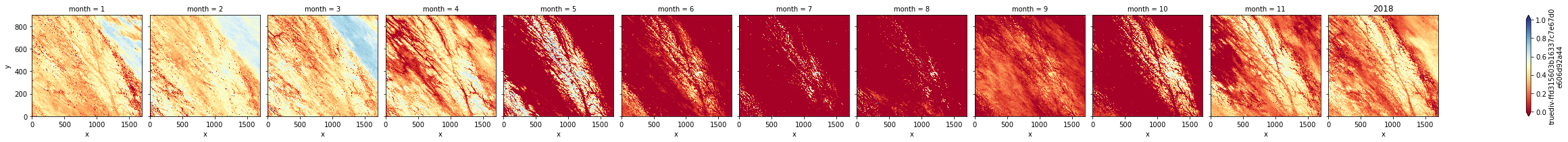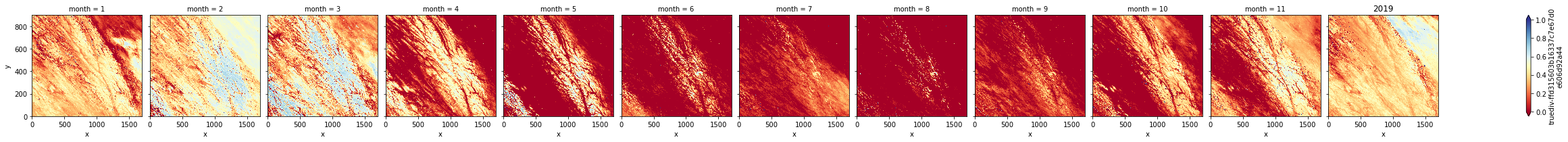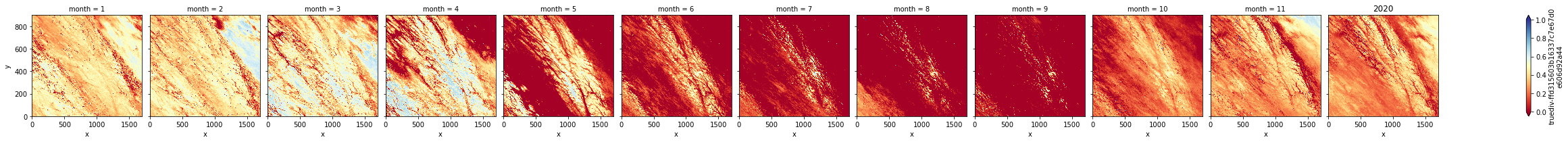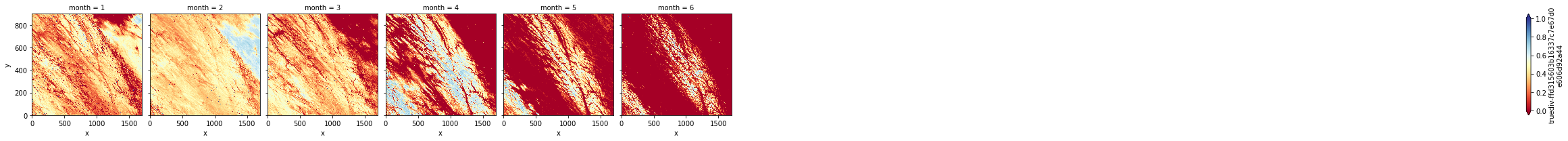# NDSI with MODIS and Dask#

This tutorial notebook demonstrates how to find, visualize, and analyze the Normalized Difference Snow Index with MODIS imagery, efficiently using Dask. The satellite we will be using is the MODIS Terra Surface Reflectance Daily Global 1km and 500m Version 6 (MOD09GA.006) which has a spatial resolution of 500-1000 meters and temporal resolution of 1 day. We will be focusing on the granules covering Northern British Columbia and most of Alberta.

What is NDSI?

The Normalized Difference Snow Index is used to detect the presence of snow in a pixel. Identifying snow cover is important for hazard mitigation, and for hydrological and weather forecasting. Snow has very high visible (Green) reflectance and very low reflectance in the shortwave infrared (SWIR), which makes it easy to differentiate snow cover from most cloud types.

How is NDSI calculated?

To calculate NDSI, the Visible Green light band and SWIR band are used in this formula:

• NDSI = (Green - SWIR) / (Green + SWIR)

In MOD09GA Version 6, Band 4 is green and Band 6 is SWIR.

What do NDSI values represent?

The NDSI values ranging from 0 to 1, higher values (NDSI > 0.4) represent snow. The MODIS products were tested with different NDSI thresholds ranging from 0.3 to 0.4 to map the snow cover over low-altitude glaciers due to its coarser resolution.

Since we want to use eight years of data for our analysis, running the computation using the cores of the machine we are running this notebook on is not ideal. Let’s connect to Dask Gateway so the clusters can run our work on Elastic Compute Cloud (EC2) machines.

For now, we will set our cluster to scale the workers to 20, because of the amount of data we will be working with.

:

import os
import glob
import re
import datetime
import requests
import sys
import time
import io
import math
import yaml
import tempfile

import distributed
import rasterio

import pandas as pd
import xarray as xr
import numpy as np
import rioxarray as rxr

from rasterio.plot import show
from urllib.request import urlopen, Request, build_opener, HTTPCookieProcessor

:

gateway = Gateway()
options = gateway.cluster_options()
cluster = gateway.new_cluster(options)

:

workers = 20
cluster.scale(workers)

:

client = cluster.get_client() #Make sure dask knows to use this cluster for computations

:

#client.wait_for_workers(workers - 27)
cluster

:

dask.config.set({"dask.comm.timeouts.tcp": "60s"})

:

<dask.config.set at 0x7ff5b0d97bb0>


## 2. Getting the MODIS Data#

In contrast to the Sentinel-2 and Landsat 8 satellite data which are publicly available for download, MODIS data is stored on NASA’s Land Processes Distributed Active Archive Center (LP DAAC) located at the USGS Earth Resources Observation and Science (EROS) Center. The data requires a NASA Earthdata Authentication with the user’s username and password. (https://e4ftl01.cr.usgs.gov/)

The main steps to retrieve the MODIS data follow as such:

1. Using python-cmr, select the desired data based on the Granule Queries.

2. Create an Earthdata Account and store credentials for authentication.

3. Using Hatfield’s CMR to access the LP DAAC website with the credentials and stream the data into an in-memory file object.

4. Assigning a temporary file name to the object and opening the file with rasterio.

### 2.1 Select the Granules#

Install the python-cmr which is a wrapper to the NASA EOSDIS Common Metadata Repository API. The package allows you to make queries on the available data.

:

! pip install python-cmr

Requirement already satisfied: python-cmr in /srv/conda/envs/notebook/lib/python3.8/site-packages (0.4.1)
Requirement already satisfied: requests in /srv/conda/envs/notebook/lib/python3.8/site-packages (from python-cmr) (2.24.0)
Requirement already satisfied: urllib3!=1.25.0,!=1.25.1,<1.26,>=1.21.1 in /srv/conda/envs/notebook/lib/python3.8/site-packages (from requests->python-cmr) (1.25.11)
Requirement already satisfied: idna<3,>=2.5 in /srv/conda/envs/notebook/lib/python3.8/site-packages (from requests->python-cmr) (2.10)
Requirement already satisfied: certifi>=2017.4.17 in /srv/conda/envs/notebook/lib/python3.8/site-packages (from requests->python-cmr) (2020.6.20)
Requirement already satisfied: chardet<4,>=3.0.2 in /srv/conda/envs/notebook/lib/python3.8/site-packages (from requests->python-cmr) (3.0.4)


Next we will set the parameters of our search to get the granules for the product “MOD09GA” of version 6, with the tile Horizontal 10 and Vertical 3 (indicated by the latitude, longitude point inside that tile) from 2016 to 2021.

:

# Time period
start_date = datetime.datetime(2016, 6, 30).date()
end_date = datetime.datetime(2021, 6, 30).date()

:

from cmr import GranuleQuery

# search for MOD09GA version 006 granules at latitude 56, longitude -120
api = GranuleQuery()
api.parameters(
short_name="MOD09GA",
version="006",
point=(-120, 51), # within Tile H10V3 - encompasses northern BC area
temporal=(f"{start_date}T00:00:00Z", f"{end_date}T23:59:59Z")
)

:

<cmr.queries.GranuleQuery at 0x7ff5b0d0d430>


Now we will retrieve all the granules possible for the queries using the .get_all() method.

:

granules = api.get_all()
len(granules)

:

1827

:

granules['links']['href']

:

'https://e4ftl01.cr.usgs.gov//DP109/MOLT/MOD09GA.006/2016.06.30/MOD09GA.A2016182.h10v03.006.2016187214710.hdf'


### 2.2 Create an EarthData Account#

• EARTHDATA_USER: '<username>'

• EARTHDATA_PASS: '<password>'

2. Run the cell below to load and pass in the credentials as a tuple to auth.

:

# Load the yaml file containing the authentication to EarthData
envvars = open('data/ED_config.yml' ,'r')
envvars.close()
auth=(secrets['EARTHDATA_USER'],secrets['EARTHDATA_PASS'])


### 2.3 Hatfield CMR#

Use the Hatfield CMR functions to safely access the granules and load them as an in-memory file.

:

from typing import Tuple, List
import requests
from html.parser import HTMLParser

def __init__(self):
super().__init__()

def handle_starttag(self, tag, attrs):
if attrs and attrs == 'href':

AUTH_HOST = 'urs.earthdata.nasa.gov'
def __init__(self, auth):
super().__init__()
self.auth = auth

# Overrides from the library to keep headers when redirected to or from
# the NASA auth host.
def rebuild_auth(self, prepared_request, response):
url = prepared_request.url
original_parsed = requests.utils.urlparse(response.request.url)
redirect_parsed = requests.utils.urlparse(url)
if (original_parsed.hostname != redirect_parsed.hostname) and redirect_parsed.hostname != self.AUTH_HOST and original_parsed.hostname != self.AUTH_HOST:
return

def get_session(auth, retries=5):
# s = requests.Session()
#r = Retry(total=retries, backoff_factor=0.1, status_forcelist=[500, 502, 503, 504])
return s

def get_stream(session, url, auth, previous_tries):
""" Traverse redirects to get the final url """

# stream = session.get(url, auth=auth, stream=True)
stream = session.get(url,
auth=auth,
allow_redirects=True,
stream=True)

# if we get back to the same url, it's time to download
if url in previous_tries or stream.status_code == 200:
return stream

if stream.status_code == 302:
# if stream.status_code != 200:
#     print(f"Error getting response {stream.status_code} {stream.text}")
previous_tries.append(url)
previous_tries)
else:
raise RuntimeError(
f"Earthdata Authentication Error {stream.status_code} {stream.text}"
)
# return stream

auth: tuple,
noauth: bool = False) -> io.BytesIO:
session = get_session(auth, retries=5)
if noauth:
stream = session.get(url, stream=True)
else:
stream = get_stream(session, url, auth, [])
buf = io.BytesIO()
for chunk in stream.iter_content(256 * 1024):
buf.write(chunk)
buf.seek(0,0)
return buf


## 3. Calculating the NDSI#

Now that we have the functions ready, let’s access the data and compute the NDSI! To efficiently get the NDVI for all the data, we must first load in the MODIS image data from NASA’s LPDAAC Center, using Hatfield’s CMR.

### 3.1 Stream and Load the MODIS Data#

Let’s run the cells below to generate the functions to lazily retrieve the data from NASA servers. The function lazy_get_data() takes in the url of an image from the list of granules, along with the Earthdata authentication and the Dask chunk sizes.

The url will be used to download the file as a temporary file using the Hatfield CMR, and opened using rasterio. Since the MODIS tiles cover a large area on Earth, we will be looking at only one portion for our NDSI analysis. The rasterio window will only read the part of the tile we specify. In this example, the window covers Southern British Columbia, near the Rocky Mountains.

We will convert the “windowed” numpy arrays from rasterio to dask arrays with the assigned chunksize, for optimal parallelization.

:

import warnings
warnings.filterwarnings("ignore", category=rasterio.errors.NotGeoreferencedWarning)

:

@dask.delayed
def lazy_get_data(url, auth, chunks={'band': 1, 'x': 2048, 'y': 2048}):

with tempfile.NamedTemporaryFile() as tmpfile:
tmpfile.write(buff.getvalue())
with rasterio.open(tmpfile.name, 'r') as modis_scene:
B4 = modis_scene.subdatasets
B6 = modis_scene.subdatasets

with rasterio.open(B4) as green, rasterio.open(B6) as swir:
# window(row_offset, col_offset, width, height)
window = rasterio.windows.Window(700, 1500, 1700, 900)

# read only the section specified by the window  and change to float type

# Convert numpy array to dask array in chunks

return (green_da, swir_da)


### 3.2 Calculate the NDSI of each granule#

With the bands ready for computation, we can now construct the main function for calculating the NDSI of each raster. The function get_NDSI() computes the NDSI using the input bands and transforms the resulting array into an Xarray DataArray with the dimensions, x and y.

:

@dask.delayed
def get_NDSI(green, swir):
# Compute the NDSI and return the resulting array into an Xarray DataArray
NDSI = (green - swir) / (green + swir)
NDSI_da = xr.DataArray(NDSI, dims=['y','x'])
#NDSI_da = xr.DataArray(NDSI)

return NDSI_da


Now, the code blocks underneath call the functions above to append the resulting Dask.delayed objects into a list, then computing and persisting the results to the cluster in parallel.

:

%%time

dates_arr = []
for file in granules[::3]:
# Add the date to the dates list
get_date = re.search('\d{4}\d{3}', url)
date = datetime.datetime.strptime(get_date.group(), '%Y%j').date()
dates_arr.append(date)


CPU times: user 40.5 ms, sys: 2.12 ms, total: 42.6 ms
Wall time: 40.5 ms

:

delayed_ndsi = []
delayed_ndsi.append(get_NDSI(band, band))

:

len(delayed_ndsi)

:

609

:

%%time
ndsi_arr = []
for i in delayed_ndsi:
ndsi_arr.append(i.persist())

CPU times: user 754 ms, sys: 43.1 ms, total: 797 ms
Wall time: 752 ms

:

progress(ndsi_arr)

:

%%time
final_ndsi_arrs = [i.compute().persist() for i in ndsi_arr]
#final_ndsi_arrs

CPU times: user 32.6 s, sys: 8.12 s, total: 40.7 s
Wall time: 2min


### 3.3 Combine the NDSI of all granules together in one DataArray#

The next step is to concatenate the list of DataArrays from the previous step with their respective dates into a Xarray DataArray.

:

final_ndsi = xr.concat(final_ndsi_arrs, dim=pd.DatetimeIndex(dates_arr, name='time'))
final_ndsi

:

<xarray.DataArray 'truediv-ffd315603b16337c7e67d0e606d92a44' (time: 609, y: 900, x: 1700)>
dask.array<concatenate, shape=(609, 900, 1700), dtype=float64, chunksize=(1, 900, 1700), chunktype=numpy.ndarray>
Coordinates:
* time     (time) datetime64[ns] 2016-06-30 2016-07-03 ... 2021-06-28
Dimensions without coordinates: y, x

## 4. Visualizing the Results#

Now, let’s visualize our NDSI in a monthly average over the 6 years. Instead of using HoloViz’s hvplot as we have done in the NDVI and NDWI with Dask notebooks, let’s use Xarray’s plotting powers to display our results.

The cell below iteratively creates a monthly mean for each year

:

years = np.arange(2016, 2022, 1)

years_list = []
for i in years:
i = final_ndsi.sel(time=final_ndsi.time.dt.year.isin(i)).groupby('time.month').mean('time')
years_list.append(i)

years_list

:

[<xarray.DataArray 'truediv-ffd315603b16337c7e67d0e606d92a44' (month: 7, y: 900, x: 1700)>
dask.array<stack, shape=(7, 900, 1700), dtype=float64, chunksize=(1, 900, 1700), chunktype=numpy.ndarray>
Coordinates:
* month    (month) int64 6 7 8 9 10 11 12
Dimensions without coordinates: y, x,
<xarray.DataArray 'truediv-ffd315603b16337c7e67d0e606d92a44' (month: 12, y: 900, x: 1700)>
dask.array<stack, shape=(12, 900, 1700), dtype=float64, chunksize=(1, 900, 1700), chunktype=numpy.ndarray>
Coordinates:
* month    (month) int64 1 2 3 4 5 6 7 8 9 10 11 12
Dimensions without coordinates: y, x,
<xarray.DataArray 'truediv-ffd315603b16337c7e67d0e606d92a44' (month: 12, y: 900, x: 1700)>
dask.array<stack, shape=(12, 900, 1700), dtype=float64, chunksize=(1, 900, 1700), chunktype=numpy.ndarray>
Coordinates:
* month    (month) int64 1 2 3 4 5 6 7 8 9 10 11 12
Dimensions without coordinates: y, x,
<xarray.DataArray 'truediv-ffd315603b16337c7e67d0e606d92a44' (month: 12, y: 900, x: 1700)>
dask.array<stack, shape=(12, 900, 1700), dtype=float64, chunksize=(1, 900, 1700), chunktype=numpy.ndarray>
Coordinates:
* month    (month) int64 1 2 3 4 5 6 7 8 9 10 11 12
Dimensions without coordinates: y, x,
<xarray.DataArray 'truediv-ffd315603b16337c7e67d0e606d92a44' (month: 12, y: 900, x: 1700)>
dask.array<stack, shape=(12, 900, 1700), dtype=float64, chunksize=(1, 900, 1700), chunktype=numpy.ndarray>
Coordinates:
* month    (month) int64 1 2 3 4 5 6 7 8 9 10 11 12
Dimensions without coordinates: y, x,
<xarray.DataArray 'truediv-ffd315603b16337c7e67d0e606d92a44' (month: 6, y: 900, x: 1700)>
dask.array<stack, shape=(6, 900, 1700), dtype=float64, chunksize=(1, 900, 1700), chunktype=numpy.ndarray>
Coordinates:
* month    (month) int64 1 2 3 4 5 6
Dimensions without coordinates: y, x]

:

for i in range(len(years_list)):
years_list[i].plot.imshow('x', 'y', col='month', vmin=0, vmax=1, col_wrap=12, cmap='RdYlBu')
plt.title(years[i]):

%%time

# Creates gif
from matplotlib.animation import FuncAnimation
import numpy as np
import matplotlib.pyplot as plt

fig, ax = plt.subplots(figsize=(8,8))

def animate(i):
ax.imshow(final_ndsi_arrs[i], cmap='RdYlBu', clim=(0,1))
ax.set_title("Date: {}".format(dates_arr[i]), fontsize=30)
ax.set_axis_off()

anim = FuncAnimation(fig, animate, frames=np.arange(len(final_ndsi_arrs)), interval=80)
anim.save('NDSI_anim_2016-366.gif', dpi=50, writer='pillow')
plt.close()

CPU times: user 2h 13min 41s, sys: 28 s, total: 2h 14min 9s
Wall time: 2h 14min 9s

:

len(final_ndsi_arrs)

:

366


Make sure to always explicitly shut down computing resources when you’re not using them!

:

cluster.close()